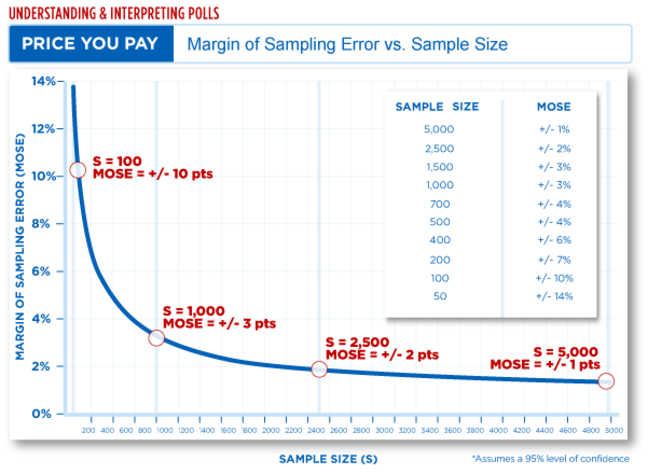3.3

The University of SheffieldHow close is a sample to the true population?

No matter how carefully data are collected, any time we take a sample from a larger population we introduce some amount of random error into the process. This is the cost of relying on a sample rather than the entire population. For polls in the media, the amount of error can be expressed by a statistic called the margin of sampling error, or MoSE for short. The MoSE is a measure of precision that tells us just how close the estimate from a sample would be to the true value from the population.

The results from any media poll can be understood within the context of repeated samples. While a single draw from the population of interest reflects one set of possible outcomes, another sample of survey respondents would likely produce slightly different results. If we were to repeat this process many times with unique samples, we can construct an interval that reveals where the true value in the population should be located.

As long as we can assume that the sample has been randomly selected - that is, there’s no systematic bias in the way that the observations were chosen - then the MoSE can be calculated using a formula (later this week, we’ll show you how you can quickly calculate the MoSE using an online calculator). In this case, the MoSE is largely determined by the sample size and our desired level of confidence in the estimate (we’ll pick this back up in a bit).

Let’s look at an example…

Perhaps the easiest way to explain the MoSE is with a specific example taken from the real world. Let’s consider the following headline from a Washington Post article: “Poll: Obama’s approval rating hits an 18-month high, is back over 50 percent”. The poll, conducted in October 2015, revealed that 51 percent of Americans approved of the way Barack Obama was handling his job as President.

Reviewing the survey methodology, we learn that the sample consisted of 1,001 American adults and corresponds to a MoSE of plus or minus 3.5 percentage points. In other words, Obama’s true job approval in the population could be as high as 54.5 percent (51 + 3.5 = 54.5) or as low as 47.5 percent (51 - 3.5 = 47.5). So, while this specific poll showed a majority of Americans approved of Obama’s job as president, the headline is a little misleading because the true level of support could be as low as 47.5 percent, which is less than a majority.

Now there’s one caveat to this interpretation: This 3.5 percent MoSE described above is valid for a 95 percent confidence interval. What exactly does that mean?

Confidence intervals

The 95 percent confidence interval can also be understood within the framework of repeated samples: In 95 out of 100 samples, the true value from the population should fall within the interval constructed by the MoSE. In our presidential job approval example, we would say that in 95 out of 100 samples the estimate of President Obama’s job approval should fall between 47.5 percent and 54.5 percent. And in the remaining 5 samples, our estimate will fall somewhere else.

If we wanted to increase our confidence that the estimate would fall within a certain range of values - let’s say for 99 out of 100 samples - then we would need to adjust our confidence interval to include a wider range of values. In general, the higher the desired level of confidence, the wider the range of values must be to accommodate this increased confidence. Usually, if a confidence interval is not specified, it is safe to assume that a 95 percent confidence level has been used.

Understanding survey size

You might have noticed that many surveys in the media contain about 1,000 survey respondents. Why is this sample size so popular among survey researchers? The short answer is that a sample of 1,000 respondents provides a nice balance between having a relatively low margin of sampling error and the added cost of collecting a larger sample. Survey research is expensive work, and as you can see from this graph, there are diminishing returns beginning around a sample size of 1,000 respondents, which corresponds to a MoSE of +/- 3 percentage points.Graph courtesy of American Association for Public Opinion Research (AAPOR)

In contrast, a sample size of only 100 respondents has too large a MoSE - nearly 10 percentage points in either direction! Yet, a sample size of 2,500 only reduces the MoSE from the original sample of 1,000 by 1 percentage point, for a total of +/- 2 percent.

Ultimately, the MoSE is important because it tells us about the precision of our estimated results relative to the true value in the population. We can actually learn a great deal about the population from a single sample but we need to be careful discussing specific point estimates as the true value; instead, we’d be better served to include some degree of uncertainty in our statements to account for sampling error.